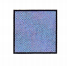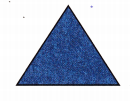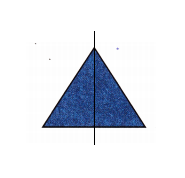# McGraw Hill Math Grade 4 Chapter 12 Lesson 8 Answer Key Line Symmetry

Practice the questions of McGraw Hill Math Grade 4 Answer Key PDF Chapter 12 Lesson 8 Line Symmetry to secure good marks & knowledge in the exams.

## McGraw-Hill Math Grade 4 Answer Key Chapter 12 Lesson 8 Line Symmetry

Solve

Look at each shape. Tell how many lines of symmetry each figure has.

Question 1.2

Explanation:
This figure have 2 lines of symmetry.

Question 2.0

Explanation:
The given figure cannot be folded in half to make equal parts
So, the figure has no lines of symmetry.

Question 3.2

Explanation:
This figure have 2 lines of symmetry.

Draw lines of symmetry on each shape if you can.

Question 4.Explanation:
I drew 1 line of symmetry on given the shape.

Question 5.Explanation:
I drew 1 line of symmetry on given the shape.

Question 6.